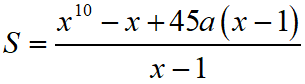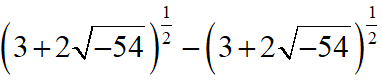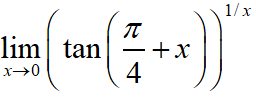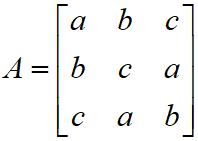Monday, September 20, 2021
Home > IIT JEE Main and Advance > JEE Main 2020 Question Paper Answer Keys Solutions 2 September Evening Shift

# JEE Main 2020 Question Paper Answer Keys Solutions 2 September Evening Shift

Hi Students, welcome to Amans Maths Blogs (AMB). On this post, you will get JEE Main 2020 Question Paper Answer Keys Solutions 2 September Evening Shift. As you know that Joint Entrance Examination means JEE Main and JEE Advanced is one of the most important entrance exam in engineering entrance examination. JEE Main 2020 is Computer Based Test (CBT) and it was conducted by the National Testing Agency (NTA). JEE Main 2020 exam duration is three hours and the exam consists of 300 marks (Maximum Marks). JEE Main 2020 Questions Paper has 2 sections in all three Subject Papers (Physics, Chemistry, Mathematics) and each subject has 25 questions.

## JEE Main 2020 Physics Questions Paper with Answer Keys & Solutions

In JEE Main 2020 Question Paper Answer Keys Solutions 2 September Morning Shift, Physics question paper’s answer keys and solutions by Resonance.

JEE Main 2020 Physics Question Paper with Answer Keys and Solutions (By Resonance)

## JEE Main 2020 Chemistry Questions Paper with Answer Keys & Solutions

In JEE Main 2020 Question Paper Answer Keys Solutions 2 September Morning Shift, Chemistry question paper’s answer keys and solutions by Resonance.

JEE Main 2020 Chemistry Question Paper with Answer Keys and Solutions (By Resonance)

## JEE Main 2020 Mathematics Questions Paper with Answer Keys & Solutions

In JEE Main 2020 Question Paper Answer Keys Solutions 2 September Evening Shift, Mathematics question paper’s answer keys and solutions by Amans Maths Blogs (AMB).

Instructions for SECTION 1 : (Maximum Marks : 80):

This section contains 20 multiple choice questions. Each question has 4 choices (1), (2), (3) and (4) for its answer, out of which Only One is correct.

JEE Main 2020 Mathematics Questions with Answer Keys and Solutions : Ques No 1

Let S be the sum of the first 9 term of the series : {x + ka} + {x2 + (k + 2)a} + {x3 + (k + 4)a} + {x4 + (k + 6)a} + …….. where a ≠ 0 and x ≠ 1.

If, then k is equal to

Options:

A. –3

B. 1

C. –5

D. 3

Solution:

JEE Main 2020 Maths Questions Answer Keys Solutions 2nd Sep Shift 2 : Ques No 2

The imaginary part ofcan be

Options:

A. √(-6)

B. √6

C. -2√6

D. 6

Solution:

JEE Main 2020 Mathematics Questions with Answer Keys and Solutions : Ques No 3

A plane passing through the point (3,1,1) contains two lines whose direction ratios are 1, – 2, 2 and 2, 3,–1 respectively. If this plane also passes through the point(α,–3,5), then α is equal to

Options:

A. 5

B. 10

C. –5

D. –10

Solution:

JEE Main 2020 Maths Questions Answer Keys Solutions 2nd Sep Shift 2 : Ques No 4

The equation of the normal to the curve y = (1 + x)2y + cos2(sin–1 x) at x = 0 is :

Options:

A. y = 4x + 2

B. y + 4x = 2

C. x + 4y = 8

D. 2y + x = 4

Solution:

JEE Main 2020 Mathematics Questions with Answer Keys and Solutions : Ques No 5is equal to

Options:

A. 2

B. 1

C. e

D. e2

Solution:

JEE Main 2020 Maths Questions Answer Keys Solutions 2nd Sep Shift 2 : Ques No 6

For some θ ∈ (0, π/2), if the eccentricity of the hyperbola, x2 – y2 sec2θ = 10 is √5 times the
eccentricity of the ellipse , x2sec2θ + y2 = 5, then the length of the latus rectum of the ellipse, is

Options:

A. 2√5 / 3

B. 2√6

C. 4√5 / 3

D. √30

Solution:

JEE Main 2020 Mathematics Questions with Answer Keys and Solutions : Ques No 7

Which of the following is a tautology ?

Options:

A. (~p) ∧ (p ∨ q) → q

B. (q → p) ∨ ~(p → q)

C. ~q ∨ (p ∧ q) → q

D. (p → q) ∧ (q → p)

Solution:

JEE Main 2020 Maths Questions Answer Keys Solutions 2nd Sep Shift 2 : Ques No 8

Let EC denote the complement of an event E. Let E1, E2 and E3 be any pairwise independent events with P(E1) > 0 and P(E1 ∩ E2 ∩ E3) = 0 then (E3C ∩ E3C/E1) is equal to

Options:

A. P(E3C) – P(E2)

B. P(E3C) – P(E2C)

C. P(E3) – P(E2C)

D. P(E2C) – P(E3)

Solution:

JEE Main 2020 Mathematics Questions with Answer Keys and Solutions : Ques No 9

Let A = {x = (x, y, z)T : PX = 0 and x2 + y2 + z2 = 1}, where,then set A

Options:

A. is a singleton.

B. contains more than two elements.

C. contains exactly two elements.

D. is an empty set.

Solution:

JEE Main 2020 Maths Questions Answer Keys Solutions 2nd Sep Shift 2 : Ques No 10

If a curve y = f(x), passing through the point (1,2), is the solution of the differential equation 2x2dy = (2xy + y2) dx, then f(1/2) is equal to

Options:

A. 1/(1 – loge2)

B. 1 + loge2

C. 1/(1 + loge2)

D. -1/(1 + loge2)

Solution:

JEE Main 2020 Mathematics Questions with Answer Keys and Solutions : Ques No 11

Let a, b, c ∈ R be all non-zero satisfy a3 + b3 + c3 = 2. If the matrixstratifies AT A = I , then a value of abc can be :

Options:

A. 1/3

B. -1/3

C. 3

D. 2/3

Solution:

JEE Main 2020 Maths Questions Answer Keys Solutions 2nd Sep Shift 2 : Ques No 12

If the equation cos4θ + sin4θ + λ = 0 has real solutions for θ, then λ lies in interval :

Options:

A. (-5/4, -1)

B. [-1, -1/2]

C. (-1/2, -1/2]

D. [-3/2, -5/4]

Solution:

JEE Main 2020 Mathematics Questions with Answer Keys and Solutions : Ques No 13

Consider a region R = {(x, y) ∈ R2 : x2 ≤ y ≤ 2x}. If a line y = α divides the area of region R into two equal parts, then which of the following is true ?

Options:

A. 3α2 – 8α + 8 = 0

B. 3α3 – 6α3/2 – 16 = 0

C. 3α3 – 6α2 + 16 = 0

D. 3α2 – 8α3/2 + 8 = 0

Solution:

JEE Main 2020 Maths Questions Answer Keys Solutions 2nd Sep Shift 2 : Ques No 14

The set of all possible values of θ in the interval (0, π) for which the points (1,2) and (sinθ, cosθ) lie on the same side of the line x + y = 1 is

Options:

A. (0, π/2)

B. (0, π/4)

C. (π/4, 3π/4)

D. (0, 3π/4)

Solution:

JEE Main 2020 Mathematics Questions with Answer Keys and Solutions : Ques No 15

Let f(x) be a quadratic polynomial such that f(–1) + f(2) = 0. If one of the roots of f(x) = 0 is 3, then its other root lies in :

Options:

A. (1, 3)

B. (-1, 0)

C. (-3, -1)

D. (0, 1)

Solution:

JEE Main 2020 Maths Questions Answer Keys Solutions 2nd Sep Shift 2 : Ques No 16

The area (in sq. units) of an equilateral triangle inscribed in the parabola y2 = 8x, with one of its vertices on the vertex of this parabola is :

Options:

A. 64√3

B. 192√3

C. 128√3

D. 256√3

Solution:

JEE Main 2020 Mathematics Questions with Answer Keys and Solutions : Ques No 17

Let f : R → R be a function which satisfies f(x + y) = f(x) + f(y) ∀ x, y ∈ R. If f(1) = 2 and, n ∈ N then the value of n, for which g(n) = 20, is

Options:

A. 9

B. 20

C. 5

D. 4

Solution:

JEE Main 2020 Maths Questions Answer Keys Solutions 2nd Sep Shift 2 : Ques No 18

If the sum of first 11 terms of an A.P. , a1, a2, a3 ….. is 0 (a1 ≠ 0), then the sum of the A.P., a1, a3, a5, ….. a23 is ka1 , where k is equal to :

Options:

A. 121/10

B. -121/10

C. -72/10

D. 72/5

Solution:

JEE Main 2020 Mathematics Questions with Answer Keys and Solutions : Ques No 19

Let n > 2 be an integer. Suppose that there are n Metro stations in a city located around a circular path. Each pair of nearest stations is connected by a straight track only. Further, each pair of nearest station is connected by blue line, whereas all remaining pairs of stations are connected by red line. If number of red lines is 99 times the number of blue lines, then the value of n is

Options:

A. 199

B. 101

C. 201

D. 200

Solution:

JEE Main 2020 Maths Questions Answer Keys Solutions 2nd Sep Shift 2 : Ques No 20

Let f : (–1, ∞) → R be defined by f(0) = 1 and f(x) = [loge(1 + x)] / x, x ≠ 0, then the function is

Options:

A. increases in (–1,0) and decreases in (0,∞).

B. decreases in (–1,∞)

C. decreases in (–1,0) and increases in (0,∞).

D. increases in (–1,∞)

Solution:

Instructions for SECTION 2 : (Maximum Marks : 20):

This section contains FIVE (05) questions. The answer to each question is NUMERICAL VALUE with two digit integer and decimal upto one digit.

If the numerical value has more than two decimal places truncate/round-off the value upto TWO decimal places.

Full Marks : +4 If ONLY the correct option is chosen

Zero Marks : 0 In all other cases

JEE Main 2020 Mathematics Questions with Answer Keys and Solutions : Ques No 21

Let the position vectors of points ‘A’ and ‘B’ be i + j + k and 2i + j + 3k, respectively. A point P divides the line segment AB internally in the ratio λ : 1 (λ > 0). If O is the origin and OB.OP – 3|OA x OP|2 = 6, then λ is equal to

Solution:

JEE Main 2020 Maths Questions Answer Keys Solutions 2nd Sep Shift 2 : Ques No 22

Let [t] denote the greatest integer less than or equal to t. Then the value ofis

Solution:

JEE Main 2020 Mathematics Questions with Answer Keys and Solutions : Ques No 23

For a positive integer n, (1 + 1/x)n, is expanded in increasing powers of x. If three consecutive
coefficients in this expansion are in the ratio, 2 : 5 : 12 , then n is equal to _______

Solution:

JEE Main 2020 Maths Questions Answer Keys Solutions 2nd Sep Shift 2 : Ques No 24

If, then dy/dx at x = 0 is

Solution:

JEE Main 2020 Mathematics Questions with Answer Keys and Solutions : Ques No 25

If the variance of the terms in an increasing A.P. b1 , b2 , b3 , …….. , b11 is 90, then the common
difference of this A.P. is